# NCERT Solutions For Class 10 Maths Chapter 13 Exercise 13.3

Go back to  'Surface Areas and Volumes'

## Chapter 13 Ex.13.3 Question 1

A metallic sphere of radius $$4.2\rm \,{cm}$$ is melted and recast into the shape of a cylinder of radius $$6\rm \,{cm}$$. Find the height of the cylinder.

### Solution

What is known?

Radius of the metallic sphere $$4.2 \,\rm{cm}$$ and radius of the cylinder $$6 \,\rm{cm}$$

What is unknown?

The height of the cylinder.

Reasoning:

Draw a figure to visualize the shapes better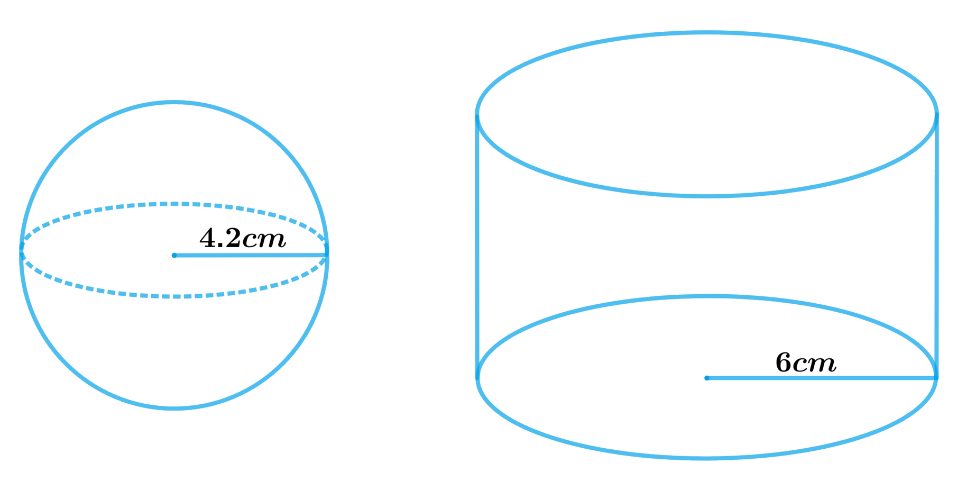Since, a metallic sphere is melted and recast into the shape of a cylinder then their volume must be same.

Volume of the sphere $$=$$ Volume of the cylinder

We will find the volume of the sphere and cylinder by using formulae;

Volume of the sphere\begin{align} = \frac{4}{3}\pi {r^3}\end{align}

where $$r$$ is the radius of the sphere

Volume of the cylinder $$= \pi {r^2}h$$

where $$r$$ and $$h$$ are radius and height of the cylinder respectively

Steps:

Radius of the hemisphere, $${r_1} = 4.2 \rm cm$$

Radius of the cylinder, $${r_2} = 6 \rm cm$$

Let the height of the cylinder be $$h$$.

Volume of sphere $$=$$ Volume of cylinder

\begin{align}\frac{4}{3}\pi r_1^3 &= \,\pi r_2^2h\\\frac{4}{3}r_1^3 &= \,r_2^2h\end{align}

\begin{align}h &= \frac{{4r_1^3}}{{3\,r_2^2}}\\&= \frac{\begin{pmatrix} 4 \times 4.2 \rm cm \times \\ 4.2 \rm cm \times 4.2cm\end{pmatrix} }{{3 \times 6 \rm cm \times 6cm}}\\&= 2.74 \rm cm\end{align}

Hence, the height of the cylinder so formed will be $$2.74\rm{ cm.}$$

## Chapter 13 Ex.13.3 Question 2

Metallic spheres of radii $$6 \,\rm{cm}$$, $$8\, \rm{cm}$$, and $$10\, \rm{cm}$$, respectively, are melted to form a single solid sphere. Find the radius of the resulting sphere.

### Solution

What is known?

Radii of three  metallic spheres are $$6\, \rm{cm}$$, $$8\, \rm{cm}$$ and $$10 \,\rm{cm}$$

What is unknown?

The radius of the resulting sphere

Reasoning:

Draw a figure to visualize the shapes better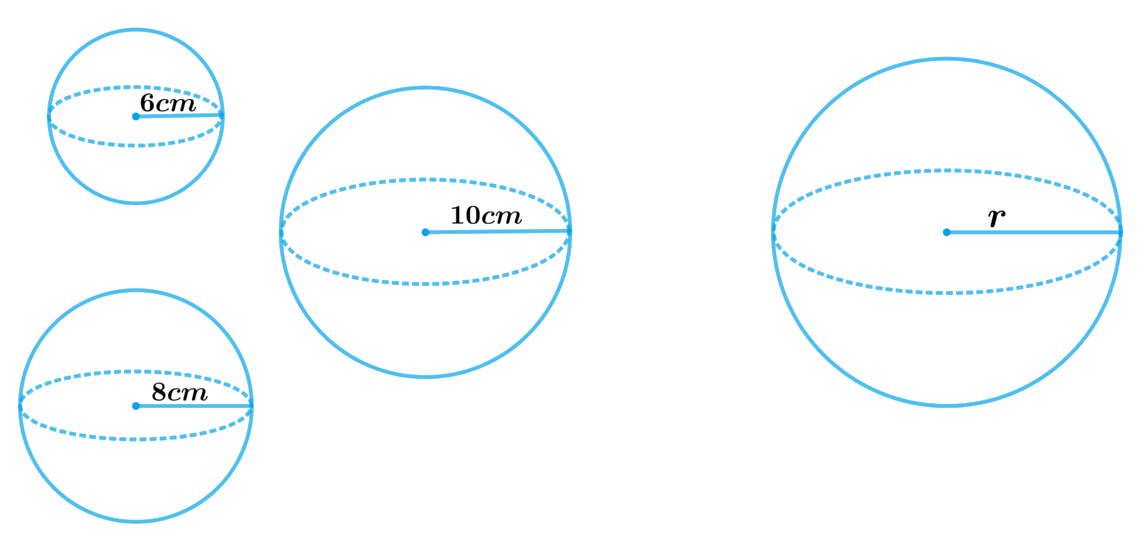Since, three metallic spheres are melted and recast into a single solid sphere then the sphere formed by recasting these spheres will be same in volume as the sum of the volumes of these spheres.

Volume of the resulting sphere $$=$$ Sum of the volumes of three spheres

We will find the volume of the sphere by using formula;

Volume of the sphere\begin{align} = \frac{4}{3}\pi {r^3}\end{align}

where $$r$$ is the radius of the sphere

Steps:

Radius of 1st sphere,$${r_1} = 6 \rm cm$$

Radius of 2nd sphere,$${r_2} = 8 \rm cm$$

Radius of 3rd sphere,$${r_3} = 10 \rm cm$$

Let the radius of the resulting sphere be $$r$$.

Volume of the resulting sphere $$=$$ Sum of the volumes of three spheres

\begin{align}\frac{4}{3}\pi {r^3} &= \frac{4}{3}\pi r_1^3 + \frac{4}{3}\pi r_2^3 + \frac{4}{3}\pi r_3^3\\ \frac{4}{3}\pi {r^3} &= \frac{4}{3}\pi \left[ {r_1^3 + r_2^3 + r_3^3} \right]\\{r^3} &= \left[ {r_1^3 + r_2^3 + r_3^3} \right]\\{r^3} &= \begin{bmatrix} \left( 6 \rm cm \right)^3 \\ + \left( 8 \rm cm \right)^3 \\+ \left( 10 \rm cm \right)^3 \end{bmatrix} \\{r^3} &= \begin{bmatrix} 216 \rm c{m^3} +\\ 512 \rm c{m^3} +\\ 1000 \rm c{m^3} \end{bmatrix} \\{r^3} &= 1728 \rm c{m^3}\\r &= 12 \rm cm\end{align}

Therefore, the radius of the sphere so formed will be $$12 \rm{cm.}$$

## Chapter 13 Ex.13.3 Question 3

A $$20 \,\rm{m}$$ deep well with diameter $$7 \,\rm{m}$$ is dug and the earth from digging is evenly spread out to form a platform $$22\, \rm{m}$$ by $$14 \,\rm{m}$$. Find the height of the platform.

[Use \begin{align}\pi \;=\;\frac{22}{7}\end{align}]

### Solution

What is known?

Depth of the well is $$20\rm{m}$$ and diameter is $$7\rm{m}$$. Length of the platform is $$22\rm{m}$$ and breadth is $$14\rm{m.}$$

What is unknown?

The height of the platform.

Reasoning:

Draw a figure to visualize the shapes better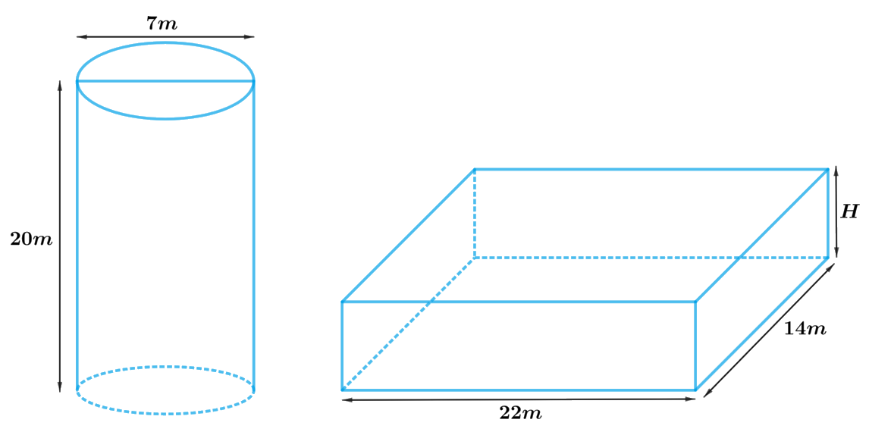The shape of the well will be cylindrical, and soil evenly spread out to form a platform will be in a cuboidal shape.

Therefore, volume of the soil dug from the well will be equal to the volume of soil evenly spread out to form a platform.

Volume of soil dug out from the well $$=$$ Volume of soil used to make such platform

Hence, Volume of the cylindrical well $$=$$ Volume of the cuboidal platform

We will find the volume of the cylinder and cuboid by using formulae;

Volume of the cylinder $$= \pi {r^2}h$$

where $$r$$ and $$h$$ are radius and height of the cylinder respectively

Volume of the cuboid $$= lbH$$

where $$l, b$$ and $$H$$ are length breadth and height of the cuboid respectively

Steps:

Depth of the cylindrical well,$$h = 20 \rm m$$

Radius of the cylindrical well,$$r = \frac{7}{2} \rm m$$

Length of the cuboidal platform,$$l = 22 \rm m$$

Breadth of the cuboidal platform,$$b = 14 \rm m$$

Let the height of the cuboidal platform $$= H$$

Volume of the cylindrical well $$=$$ Volume of the cuboidal platform

\begin{align}\pi {r^2}h &= lbH\\H &= \frac{{\pi {r^2}h}}{{lb}}\\&= \frac{{ \begin{bmatrix} \frac{{22}}{7} \times \frac{7}{2} \rm m \times\\ \frac{7}{2} \rm m \times 20 \rm m \end{bmatrix} }}{{22 \rm m \times 14m}}\\&= \frac{5}{2} \rm m\\&= \,2.5 \rm m\end{align}

Therefore, the height of such platform will be $$2.5 \rm{m}$$

## Chapter 13 Ex.13.3 Question 4

A well of diameter $$3\,\rm{m}$$ is dug $$14\,\rm{m}$$ deep. The earth taken out of it has been spread evenly all around it in the shape of a circular ring of width $$4\,\rm{m}$$ to form an embankment. Find the height of the embankment.

### Solution

What is known?

Depth of the well is $$14\rm{m}$$ and diameter is $$3\rm{m}$$. Width of the circular ring of the embankment is $$4\rm{m}$$.

What is unknown?

The height of the embankment.

Reasoning:

Draw a figure to visualize the shapes better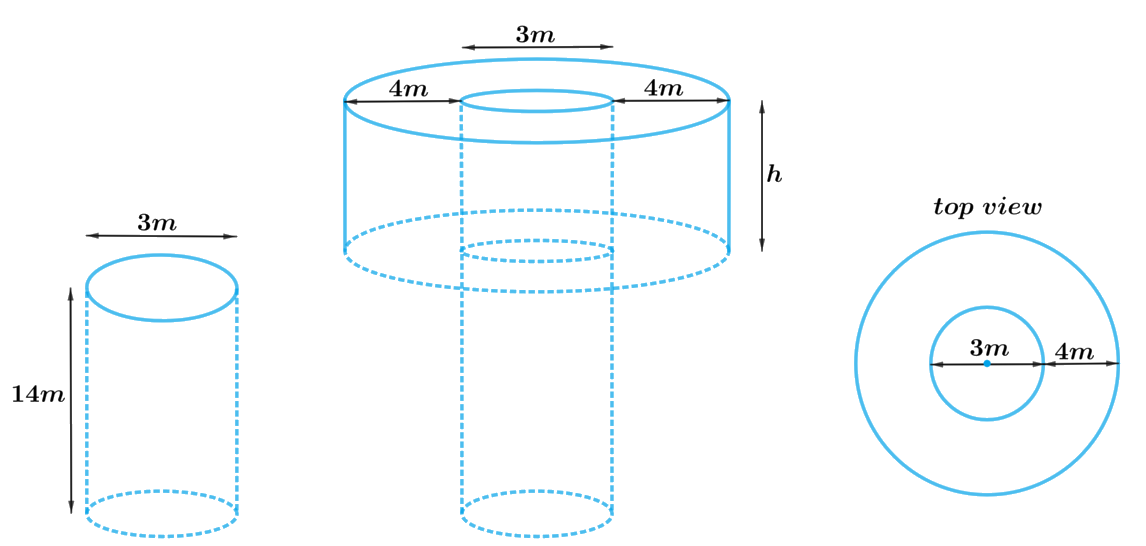From the figure, it can be observed that the shape of the well will be cylindrical, and earth evenly spread out to form an embankment around the well in a circular ring will be in a cylindrical shape (Hollow cylinder) having outer and inner radius.

Volume of the earth taken out from well $$=$$ Volume of the earth used to form embankment

Hence, Volume of the cylindrical well $$=$$ Volume of the hollow cylindrical embankment

We will find the volume of the hollow cylindrical embankment by subtracting volume of inner cylinder from volume of the outer cylinder.

Volume of the cylinder $$= \pi {r^2}h$$

where $$r$$ and $$h$$ are the radius and height of the cylinder respectively.

Steps:

Depth of the cylindrical well,$${h_1} = 14 \rm m$$

Radius of the cylindrical well,\begin{align}r = \frac{3}{2} \rm m = 1.5m\end{align}

Width of embankment $$= 20 \rm m$$

Inner radius of the embankment,\begin{align}r = \frac{3}{2} \rm m\end{align}

Outer radius of the embankment, R $$=$$ Inner radius $$+$$ Width

\begin{align}R &= 1.5 \rm m + 4m\\&= 5.5 \rm m\end{align}

Let the height of embankment be $$h$$

Volume of the cylindrical well $$=$$ Volume of the hollow cylindrical embankment

\begin{align}\pi {r^2}{h_1} &= \pi {R^2}h - \pi {r^2}h\\\pi {r^2}{h_1} &= \pi h\left( {{R^2} - {r^2}} \right)\\{r^2}{h_1} &= h\left( {R - r} \right)\left( {R + r} \right)\\h &= \frac{{{r^2}{h_1}}}{{\left( {R - r} \right)\left( {R + r} \right)}}\\&= \frac{{{{\left( {1.5 \rm m} \right)}^2} \times 14 \rm m}}{{ \begin{bmatrix}\left( {5.5\rm m - 1.5m} \right) \\ \left( {5.5 \rm m + 1.5} \right) \end{bmatrix}}}\\&= \frac{{2.25\rm {m^2} \times 14m}}{{4 \rm m \times 7m}}\\&= 1.125 \rm m\end{align}

Therefore, the height of the embankment will be $$1.125\rm{ m.}$$

## Chapter 13 Ex.13.3 Question 5

A container shaped like a right circular cylinder having diameter $$12\,\rm{cm}$$ and height $$15\,\rm{cm}$$ is full of ice cream. The ice cream is to be filled into cones of height $$12\,\rm{cm}$$ and diameter $$6\,\rm{cm}$$, having a hemispherical shape on the top. Find the number of such cones which can be filled with ice cream.

### Solution

What is known?

Diameter of the right circular cylinder is $$12\rm{cm}$$ and height is $$15\rm{cm.}$$

Diameter of the cone is $$6\rm{cm}$$ and height is $$12\rm{cm.}$$

What is unknown?

The number of cones which can be filled with ice cream.

Reasoning:

Draw a figure to visualize the shapes better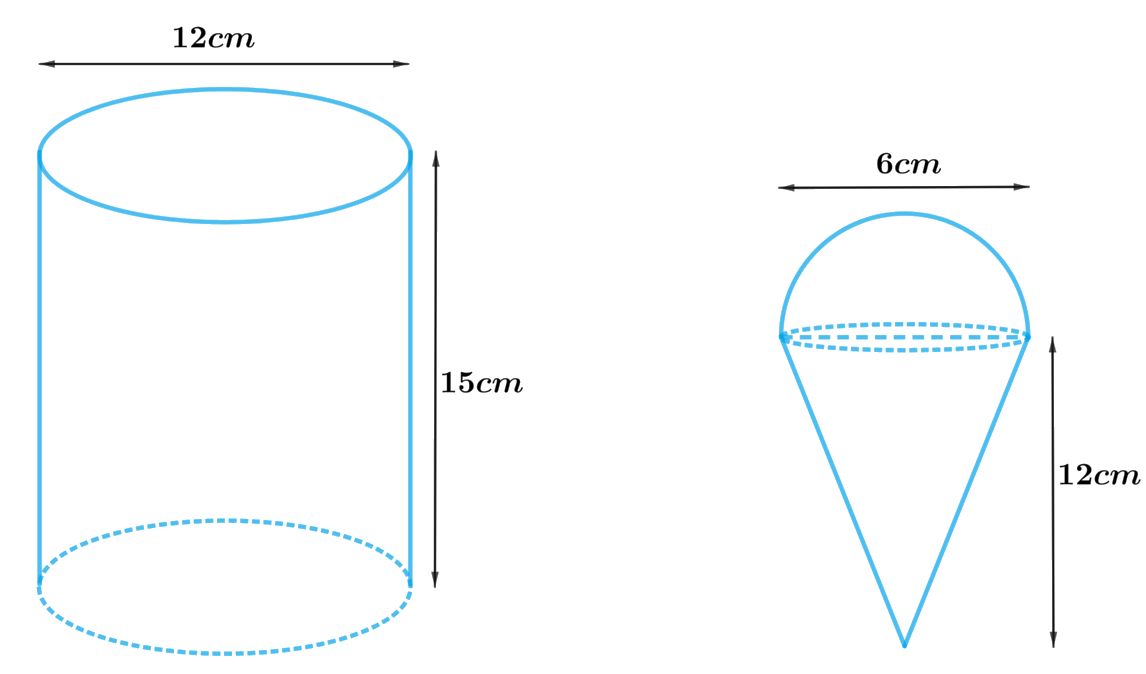From the figure it’s clear that diameter of the hemisphere is same as the cone.

Since, the ice cream is to be filled into cones having a hemispherical shape on the top the volume of ice cream filled into the cone includes volume of the cone and volume of the hemisphere.

volume of the ice cream in each cone $$=$$ volume of the cone $$+$$ volume of the hemisphere

Since, the ice cream is to be filled from a cylindrical container, the volume of the ice cream filled into cones will be same as the volume of the ice cream in cylindrical container

volume of the ice cream in cylindrical container $$=$$ number of cones filled with ice cream $$\times$$ volume of the ice cream in each cone

Hence, number of cones filled with ice cream $$=$$ volume of the ice cream in cylindrical container $$\div$$volume of the ice cream in each cone

We will find the volume of the ice cream by using formulae;

Volume of the hemisphere\begin{align} = \frac{2}{3}\pi {r^3}\end{align}

where $$r$$ is the radius of the hemisphere

Volume of the cone\begin{align} = \frac{1}{3}\pi {r^2}h\end{align}

where $$r$$ and $$h$$ are the radius and height of the cone respectively

Volume of the cylinder$$= \pi {r^2}h$$

where $$r$$ and $$h$$ are radius and height of the cylinder respectively.

Steps:

Height of cylindrical container,$$H = 15 \rm m$$

Radius of cylindrical container,\begin{align} R = \frac{{12 \rm m}}{2} = 6 \rm m\end{align}

Radius of the ice-cream cone $$=$$ Radius of the hemispherical top\begin{align} = r = \frac{{6 \rm m}}{2} = 3 \rm m\end{align}

Height of the ice-cream cone,$$h = 12 \rm m$$

Let $$n$$ ice-cream cones be filled with ice-cream of the container.

volume of the ice cream in container $$= n \times$$volume of the ice cream in each cone

\begin{align}\pi {R^2}H &= n \times \begin{pmatrix} \frac{1}{3}\pi {r^2}h \\ + \frac{2}{3}\pi {r^3} \end{pmatrix}\\ \pi {R^2}H &= n\pi \left( {\frac{1}{3}{r^2}h + \frac{2}{3}{r^3}} \right)\\{R^2}H &= \frac{1}{3}n{r^2}\left( {h + 2r} \right)\\n &= \frac{{3{R^2}H}}{{{r^2}\left( {h + 2r} \right)}}\\\, &= \frac{{ \begin{bmatrix} 3 \times {{\left( {6 \rm cm} \right)}^2} \\ \times 15 \rm cm \end{bmatrix} }}{{ \begin{bmatrix}\left( 3 \rm cm \right)^2 \times \\ \begin{pmatrix} 12 \rm cm +2 \\ \times 3 \rm cm \end{pmatrix} \end{bmatrix} }}\\&= \frac{{3 \times 36 \rm c{m^2} \times 15 \rm cm}}{{9 \rm c{m^2} \times 18 cm}}\\&= 10\end{align}

Therefore, $$10$$ ice-cream cones can be filled with the ice-cream in the container.

## Chapter 13 Ex.13.3 Question 6

How many silver coins, $$1.75\, \rm{cm}$$ in diameter and of thickness $$2\, \rm{mm}$$, must be melted to form a cuboid of dimensions  $$5.5\,\rm{cm}$$ $$\times$$ $$10\,\rm{cm}$$ $$\times$$ $$3.5\,\rm{cm}$$?
[Use \begin{align} \pi =\;\frac{22}{7}\end{align}]

### Solution

What is known?

Diameter of the coin is $$1.75\rm{cm}$$ and thickness is $$2\,\rm{mm.}$$

Dimensions of the cuboid is $$5.5 \rm cm \times 10cm \times 3.5cm$$

What is unknown?

The number of silver coins.

Reasoning:

Draw a figure to visualize the shapes better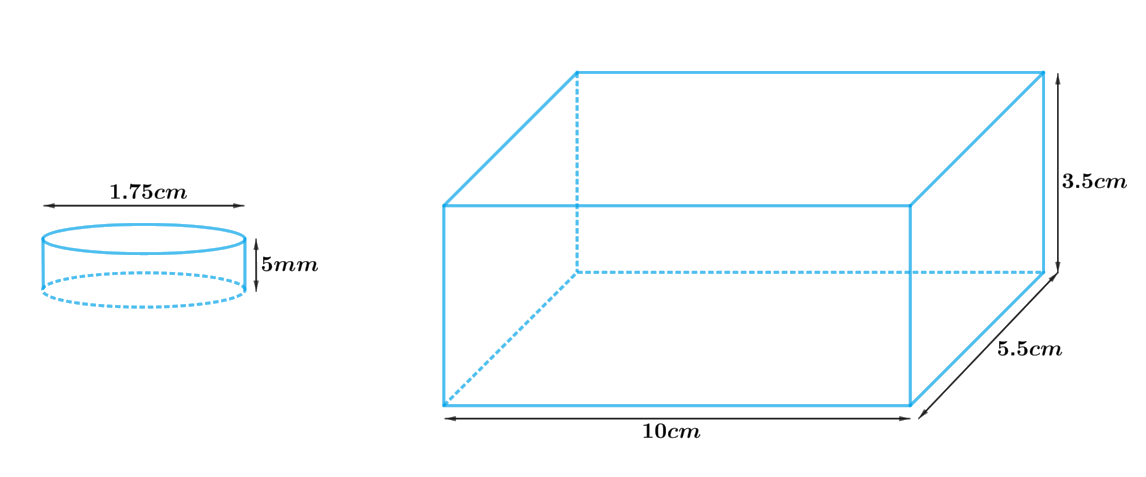From the figure it’s clear that the shape of the silver coin is cylindrical.

Since, the silver coins to be melted and recast into a single solid cuboid then the cuboid formed by recasting these coins will be same in volume as the sum of the volumes of these coins.

Sum of the volumes of the silver coins $$=$$ Volume of the cuboid

Therefore,

Number of the coins $$\times$$ volume of each coin $$=$$ volume of the cuboid

We will find the volume of the solid by using formulae;

Volume of the cuboid $$= lbh$$

Where $$l, b$$ and $$h$$ are length, breadth and height of the cuboid respectively.

Volume of the cylinder $$= \pi {r^2}{h_1}$$

where $$r$$ and $$h_1$$ are radius and height of the cylinder respectively.

Steps:

Dimensions of the cuboid,

$$l \times b \times h =\begin{Bmatrix} 5.5 \rm cm \times 10cm \\ \times 3.5 \rm cm \end{Bmatrix}$$

Height of the cylindrical coin,\begin{align} {h_1} = 2mm = \frac{2}{{10}} \rm cm = 0.2{\rm{ }}cm\end{align}

Radius of the cylindrical coin,\begin{align}r = \frac{{1.75 \rm cm}}{2} = {\rm{ }}0.875\, \rm cm\end{align}

Let $$n$$ coins be melted to form the required cuboid.

Volume of $$n$$ coins $$=$$ Volume of cuboids

\begin{align}n \times \pi {r^2}{h_1} &= l \times b \times h\\n &= \frac{{l \times b \times h}}{{\pi {r^2}{h_1}}}\end{align}

\begin{align}&= \frac{{ \begin{bmatrix} 5.5 \rm cm \times 10 \rm cm \times 3.5 \rm cm \end{bmatrix} }}{{ \begin{bmatrix} \frac{{22}}{7} \times {{(0.875 \rm cm)}^2} \times 0.2 \rm cm \end{bmatrix}}}\\&= \frac{{ \begin{bmatrix} 5.5 \rm cm \times 10 \rm cm \times 3.5 \rm cm \end{bmatrix} }}{{ \begin{bmatrix} \frac{{22}}{7} \times {{(0.875 \rm cm)}^2} \times 0.2 \rm cm \end{bmatrix} }}\\&= \frac{{ \begin{bmatrix} 5.5 \rm cm \times 10 \rm cm \times\\ 3.5 \rm cm \times 7 \end{bmatrix} }}{{ \begin{bmatrix} 22 \times 0.875 \rm cm \times 0.875 \rm cm \times \\ 0.2 \rm cm \end{bmatrix} }}\,\, \\&= 400\end{align}

Therefore, the number of coins melted to form such a cuboid is $$400$$.

## Chapter 13 Ex.13.3 Question 7

A cylindrical bucket, $$32\,\rm{cm}$$ high and with radius of base $$18\,\rm{cm}$$, is filled with sand. This bucket is emptied on the ground and a conical heap of sand is formed. If the height of the conical heap is $$24\,\rm{cm}$$. Find the radius and slant height of the heap.

### Solution

What is known?

Height of the cylindrical bucket is $$32\rm{cm}$$ and radius is $$18\rm{cm.}$$

Height of the conical heap is $$24\rm{cm}$$

What is unknown?

The radius and slant height of the heap.

Reasoning:

Draw a figure to visualize the shapes better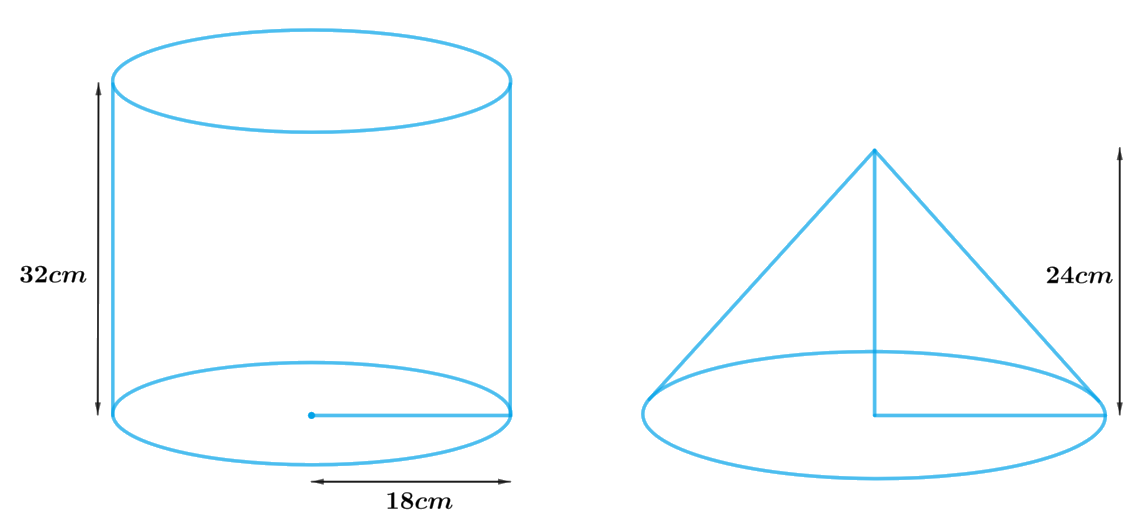Since, a cylindrical bucket filled with sand, emptied on the ground and a conical heap of sand is formed. Then the volume of sand in the cylindrical bucket will be same as the volume the conical heap of sand.

Therefore, volume the conical heap of sand $$=$$ volume of sand in the cylindrical bucket

We will find the volume of the sand by using formulae;

Volume of the cylinder $$= \pi {r^2}h$$

where $$r$$ and $$h$$ are the radius and height of the cylinder respectively

Volume of the cone \begin{align}= \frac{1}{3}\pi {r^2}h\end{align}

$l = \sqrt {{r^2} + {h^2}}$

where $$r, h$$ and $$l$$ are radius, height and slant height of cone respectively.

steps:

Height of the cylindrical bucket,$${h_1} = 32 \rm cm$$

Radius of the cylindrical bucket,$${r_1} = 18 \rm cm$$

Height the of conical heap, $$h = 24{\rm{ cm}}$$

Let the radius of the conical heap be $$r$$ and slant height be $$l.$$

volume the conical heap of sand $$=$$ volume of sand in the cylindrical bucket

\begin{align}\frac{1}{3}\pi {r^2}h &= \pi {r_1}^2{h_1}\\\frac{1}{3}\pi {r^2}h &= \pi {r_1}^2{h_1}\\ {r^2} &= \frac{{3\pi {r_1}^2{h_1}}}{h}\\{r^2} &= \frac{{ \begin{Bmatrix} 3 \times {{\left( {18 \rm cm} \right)}^2} \times \\ 18 \rm cm \times 32 \rm cm \end{Bmatrix} }}{{24 \rm cm}}\\r &= \sqrt {{{\left( {18 \rm cm} \right)}^2} \times 4} \\r &= 18 \rm cm \times 2\\\,\,r\, &= 36\,\rm cm\end{align}

Slant height, $$l = \sqrt {{r^2} + {h^2}}$$

\begin{align}&= \sqrt {{{\left( {36 \rm cm} \right)}^2} + {{\left( {24 \rm cm} \right)}^2}} \\&= \sqrt {1296 \rm c{m^2} + 576 \rm c{m^2}} \\&= \sqrt {1872 \rm c{m^2}} \\&= 12\sqrt {13} \rm cm\end{align}

Therefore, the radius and slant height of the conical heap are $$36\,\rm{cm}$$ and $${\text{12}}\sqrt {{\text{13}}\,} \,{\text{cm}}$$  respectively.

## Chapter 13 Ex.13.3 Question 8

Water in canal, $$6\,\rm{m}$$ wide and $$1.5\,\rm{m}$$ deep, is flowing with a speed of $$10\,\rm{km/h}$$. how much area will it irrigate in $$30$$ minutes, if $$8\,\rm{cm}$$ of standing water is needed?

### Solution

What is known?

Width of the canal is $$6\rm{m}$$ and depth is $$1.5\rm{m}$$ and speed of flowing water is $$10\rm{km/h}$$

Height of the standing water is $$8\rm{cm}$$ in the area to be irrigated for $$30$$ minutes.

What is unknown?

The area to be irrigated in $$30$$ minutes with $$8\rm{cm}$$ of standing water.

Reasoning:

Draw a figure to visualize the shapes better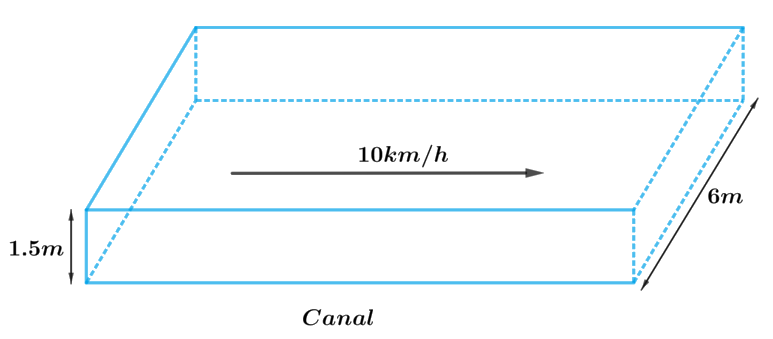From the figure it’s clear that shape of the cross-section of the canal is cuboidal. So, volume of the water, flowing at the speed of $$10\rm{km/h}$$ for $$30$$ minutes will be same as the volume of water to irrigate the area with $$8\rm{cm}$$ of standing water.

To find the volume of the water we need to find length of the water flowing through canal in $$30$$ minutes at the speed of $$10\rm{km/h}$$

Hence, length of the water flowing through canal in $$1$$ hour $$= 10\rm{km}$$

Length of the water flowing through canal in $$30$$ minutes $$= 5\rm{km} = 5000\rm{m}$$

We will find the volume of the water by using formula;

Volume of the cuboid $$= lbh$$

where $$l, b$$ and $$h$$ are length, breadth and height of the cuboid respectively.

Volume of the water to irrigate the area $$=$$ Area to be irrigated $$\times$$ height of the standing water

Volume of water flowing through the canal in $$30$$ minutes $$=$$ Area to be irrigated $$\times$$ height of the standing water

Width of the cuboidal canal, $$b = 6 \rm m$$

Depth of the cuboidal canal,$$h = 1.5 \rm m$$

Speed of water flowing through the canal is $$10\rm{km/h}$$

Length of the water flowing through canal in $$1$$ hour $$(60\rm{minutes)}= 10\rm{km}$$ Length of the water flowing through canal in $$30$$ minutes\begin{align} = \frac{{30}}{{60}} \times 10 \rm km = 5km\end{align}

$l = 5 \times 1000 \rm m = 5000m$

Height of the standing water,\begin{align}{h_1} = 8 \rm cm = \frac{8}{{100}}m = 0.08m\end{align}

Volume of water flowing through the canal in$$30\,\rm{minutes} =$$ Area to be irrigated  $$\times$$ height of the standing water

$$lbh =$$ Area to be irrigated $$\times {\rm{ }}{h_1}$$

Area to be irrigated \begin{align}= \frac{{lbh}}{{{h_1}}}\end{align}

\begin{align}&= \frac{{5000 \rm m \times 6m \times 1.5m}}{{0.08 \rm m}}\\&= 562500 \rm {m^2}\end{align}

Therefore, area irrigated in $$30$$ minutes is $$562500\text{ m}^2.$$

## Chapter 13 Ex.13.3 Question 9

A farmer connects a pipe of internal diameter $$20\,\rm{cm}$$ from a canal into a cylindrical tank in her field, which is $$10\,\rm{m}$$ in diameter and $$2\,\rm{m}$$ deep. If water flows through the pipe at the rate of $$3\,\rm{km/h}$$, in how much time will the tank be filled?

### Solution

What is known?

Internal diameter of the pipe is $$20\,\rm{cm}$$ and speed of water flows through the pipe is $$3\,\rm{km/h.}$$

Diameter of the cylindrical tank is $$10\rm{m}$$ and depth is $$2\,\rm{m}$$

What is unknown?

Time taken to fill the tank.

Reasoning:

Draw a figure to visualize the shapes better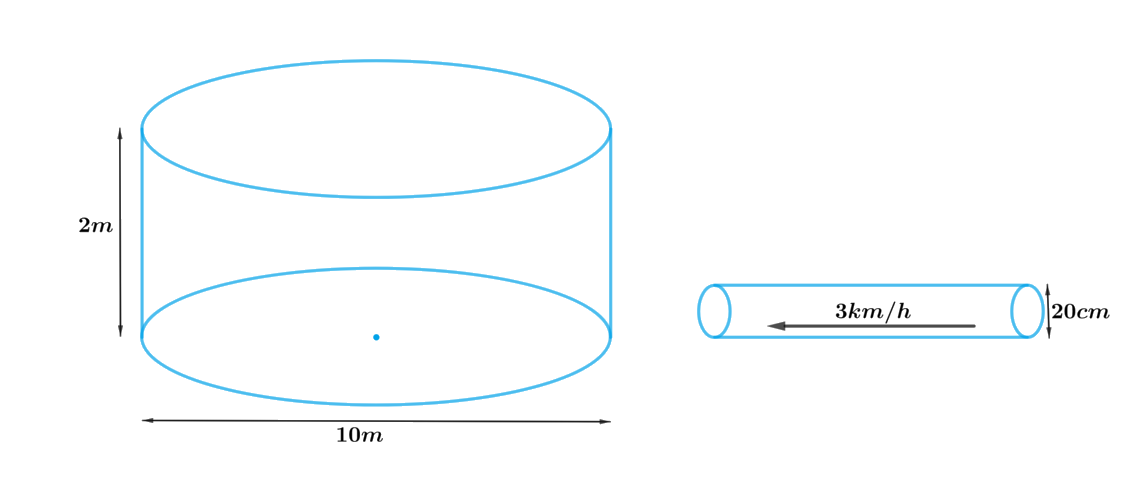From the figure it’s clear that shape of the cross-section of the pipe is cylindrical. So, volume of the water, flowing at the speed of $$3\,\rm{km/h}$$ through the pipe to fill the tank will be same as the volume of water in the cylindrical tank.

To find the volume of the water we need to find length of the water flowing through the pipe at the speed of $$3\,\rm{km/h}$$

Hence, length of the water flowing through the pipe in $$1\rm{hour(60minutes)} = 3\rm{km}$$

Length of the water flowing through the pipe in $$1\rm{minute} = 50\rm{m}$$

We will find the volume of the water by using formula;

Volume of the cylinder $$= \pi {r^2}h$$

where $$r$$ and $$h$$ are radius and height of the cylinder respectively.

Therefore,

Volume of water flowing through the pipe $$=$$ volume of water in the cylindrical tank

Steps:

Radius of the cylindrical tank,\begin{align} R = \frac{{10 \rm m}}{2} = 5 \rm m\end{align}

Depth of the cylindrical tank,$$H = 2 \rm m$$

Radius of the cylindrical pipe,\begin{align} r = \frac{{20 \rm cm}}{2} = \frac{{10}}{{100}} \rm m = 0.1m\end{align}

Length of the water flowing through the pipe in $$1\rm{hour (60minutes)} = 3\rm{km}$$

Length of the water flowing through the pipe in $$1\rm{minute,}$$\begin{align} h = \frac{{3 \rm km}}{{60}} = \frac{{3 \times 1000 \rm m}}{{60}} = 50 \rm m\end{align}

Let the tank be filled completely in $$t$$ minutes.

Volume of water flowing through pipe in $$t$$ minutes $$=$$ volume of water in cylindrical tank

\begin{align}t \times \pi {r^2}h &= \pi {R^2}H\\t &= \frac{{{R^2}H}}{{{r^2}h}}\\&= \frac{{5 \rm m \times 5 \rm m \times 2 \rm m}}{{0.1 \rm m \times 0.1 \rm m \times 50 \rm m}}\\&= 100\end{align}

Therefore, the cylindrical tank will be filled in $$100$$ minutes.